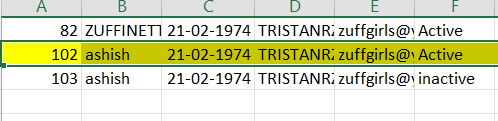# How to get row with highest value in row 0 and active in last row

abc.XLSX (8.5 KB)
in the final output I wantthis yellow part as a result.

Hey

give a try with

Left Assign drResult (Type DataRow)

``````dtInput.AsEnumerable().Where(Function(r) r(5).ToString().ToUpper().Equals("ACTIVE")).OrderByDescending(Function(x) CInt(x(0))).FirstOrDefault()
``````

Regards

its works, but I want the result in data table form because I have to write the result in another write range.

Hey

just change it a little bit, kindly try this
LeftSide yourDTResult

``````dtInput.AsEnumerable().Where(Function(r) r(5).ToString().ToUpper().Equals("ACTIVE")).OrderByDescending(Function(x) CInt(x(0))).Take(1).CopyToDataTable
``````

Regards!

`dtInput=dtInput.AsEnumerable().Where(Function(r) r(5).ToString().ToUpper().Equals("ACTIVE")).OrderByDescending(Function(x) CInt(x(0))).FirstOrDefault().CopyToDataTable()`

Regards,
Arivu

1 Like

ThankYouCan you please tell me how to learn this type of code?